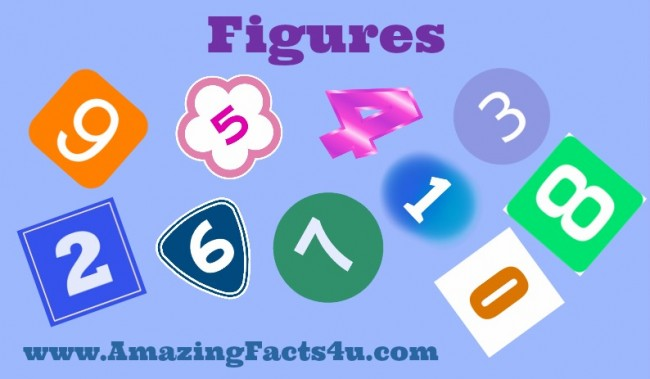# Figures#### 20 Amazing and surprising Facts About Figures | Amazing Facts 4U

1. 111, 111, 111 X 111, 111, 111 = 12, 345, 678, 987, 654, 321   Amazing!
2. Amazingly you can get the sum of hundred using all digits in succession in various ways.  (A) 12+3-4+5+67+8+9 = 100 , (B)  123 – 45 – 67 + 89 = 100  ,(C) 123 + 4 – 5 + 67 – 89 = 100  ,(D) 123 – 4 – 5 – 6 – 7 + 8 – 9 = 100  , (E) 1 + 23 – 4 + 5 + 6 + 78 – 9 = 100.
3. Fact is 2,520 is the smallest number that can be exactly divided by all the numbers 1 to 10.
4. 142857 is an amazing cyclic number, i.e., its digits are rotated around when multiplied by any number from 1 to 6.
5. 1729 is the only number that is the sum of 2 cubes in two different ways. 10^3 + 9^3 = 1729 & 12^3 + 1^3 = 1729. It is named after Ramanujan , an Indian mathematician.
6. This amazing equation uses all the digits from 0 to 9.  69^3 = 328509.
7. The amazing number 1274953680 uses all the digits 0-9 and you can divide it exactly by any number from 1-16.
8. There is a way of amazingly writing 1 by using all ten single-digit numbers only once: 148/296 + 35/70 = 1.
9. 1^2 + 2^2 + 3^2 + 4^2 + 5^2 +… +22^2 + 23^2 + 24^2 = 70^2   Amazing !
10. The fact is that the product of any four consecutive numbers is divisible by 24!
11. Multiply 37,037 by any single number (1-9), then multiply that number by 3.  Amazingly every digit in the answer will be the same as that first single number.e.g. 37,037 *2*3 = 222,222.
12. Amazing fact is that there are just four numbers (after 1) which are the sums of the cubes of their digits. These are 153 , 370 , 371 & 407.
13. If you multiply 21978 by 4 it amazingly turns backwards. 21978 x 4 = 87912.
14. 1089 multiplied by 9 amazingly gives an exact reverse: 9801. This also works with 10989 or 109989 or 1099989 and so on.
15. 13^2 = 169 and if you write both numbers backwards you get 31^2 = 961. This also works with 12 because 12^2 = 144 and 21^2 =441.
16. 1 ÷ 37 = 0·0270270270… and 1 ÷ 27 = 0·0370370370. Amazing coincidence !
17. 1/1089 = 0·00091827364554637281  .  Amazingly this is table of nine.
18. To multiply 10,112,359,550,561,797,752,808,988,764,044,943,820,224,719 by 9 you just move the 9 at the very end up to the front. Amazingly it’s the only number that does this.
19. 3,608,528,850,368,400,786,036,725 has 25 digits and divides by 25. But amazingly if you just take the first n digits, the result will divide by n. For example, 360852 are the first 6 digits and 360852 divides by 6.
20. Amazing fact is that any 2-digit number multiplied by 11 is a 3-digit number where the middle digit is the sum of other two, like: 21 × 11 = 231 (2+1=3) ,13 × 11 = 143 (1+3=4) ,44 × 11 = 484 (4+4=8) … etc.